Chapter Notes: Real Numbers

# Real Numbers Chapter Notes - Mathematics (Maths) Class 10

 Table of contentsReal NumbersClassification of Real NumbersFundamental Theorem of ArithmeticRevisiting Irrational NumbersEuclid’s Division Lemma (Old Syllabus)Euclid’s Division Algorithm (Old Syllabus)Revisiting Rational Numbers and their Decimal Expansion (Old Syllabus)## Real Numbers

All rational and irrational numbers are called real numbers. E.g. 3, 4,√5  , 8/9 . The set of real numbers is denoted by R. Real Numbers can be represented on a number line.

##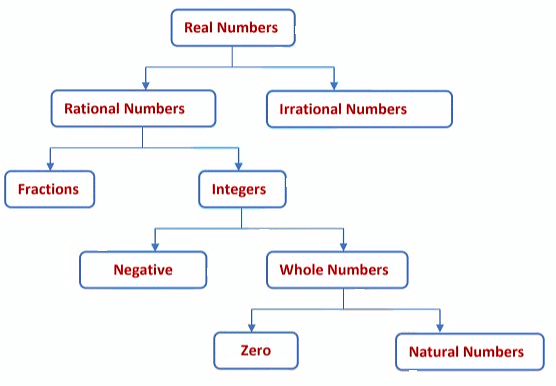Natural Numbers

Natural Numbers is a set of counting numbers. They are denoted by N.
N = { 1, 2, 3, 4..........∞}

### Whole Numbers

Whole numbers is a set of natural numbers plus zero.
W = {0, 1, 2, 3 ......... ∞}

### Integers

Integers is a set of whole numbers and negative of all natural numbers.
Z = { -3, -2, -1, 0, 1, 2, 3 }

### Rational Numbers

All the numbers that can be written in the form , where and are integers and ≠ 0 are called rational numbers.

E.g. 8/11, -3/17

### Irrational Numbers

All the numbers that cannot be written in the form , are called irrational numbers. All the non-terminating and non-repeating decimal numbers are irrational numbers.

E.g. √5, √3 , √5 + √3, π

• Now, consider √ . When we calculate the value of √3 we get √3  =1.7320508075688  here the decimal number do not repeat and do not terminate. Hence, it is an irrational number.
• √3  is an irrational number, but √ is not an irrational number because √9 = 3 , which is a rational number.
Hence, the square roots of all the numbers do not give an irrational number.
• The value of the famous irrational number π is 3.145926...... Again the decimal number is neither repeating nor terminating.

Question for Chapter Notes: Real Numbers
Try yourself:Which of the following numbers is NOT an irrational number?

## Fundamental Theorem of Arithmetic

To understand the fundamental theorem of Arithmetic first it is important to know, what are composite numbers and prime numbers.

### Composite Number

Composite Numbers are those numbers that have at least one factor other than 1 and the number itself.
Consider a number, 10. Now the factors of 10 are 1, 2, 5 and 10. So it is a composite number.

### Prime Number

Prime Numbers are those numbers which have exactly two factors, 1 and the number itself.
Let us take one more number 23. Now the factors of 23 are 1 and 23. That means it has two factors 1 and the number itself, so it is called a  prime number.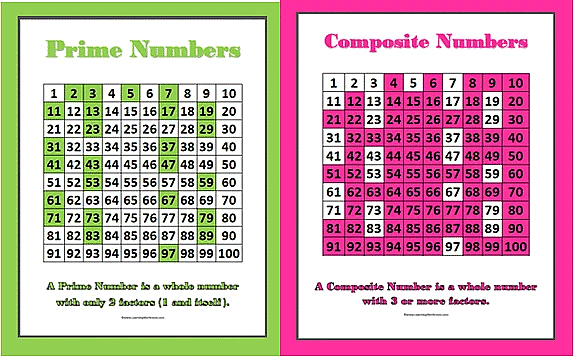Prime and Composite Numbers

Theorem:  Fundamental Theorem of Arithmetic states that every composite number can be expressed as a product of primes, and this factorization is unique, apart from the order in which the prime factors occur.

Any composite number can be written as a product of primes in one way only as long as we are not particular about the order in which the primes occur.
Let us consider an example here, we will use a tree diagram to show the  factors of 270.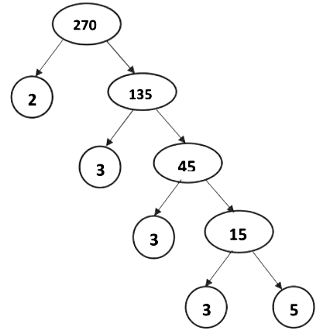270 = 2 x 33 x 5
270 = 50 x 33 x 2
Here, in the prime factorization of 270, the prime numbers appearing in both the cases are the same only the order in which they appear are different.
Therefore, the prime factorization of 270 is unique except for the order in which the primes occur.

Example: Check whether 15n can end with digit zero for any natural number n.

Sol:

If a number ends with the digit 0, then it is divisible by both 2 and 5.
But prime factors of 15 are 3 and 5.
15n = (3 × 5)n = 3n × 5n
Here, the prime factorization of 15n contains only 5 but not 2.
The uniqueness of the Fundamental Theorem of Arithmetic guarantees that there are no other primes in the prime factorization of 15n.
Therefore,15n cannot end with digit zero for any natural number n.

Example: Explain, why (7 × 6 × 5 × 4 × 3 × 2 × 1) + 5 and (3 × 5 × 13 × 46) + 23 is a composite number?

Sol:

Composite numbers are those numbers that have at least one factor other than 1 and the number itself. E.g.4, 6, 9
(i) (7 × 6 × 5 × 4 × 3 × 2 × 1) + 5 = 5040 + 5 = 5045
5045 = 5 × 1009
As the factors of 5045 are 5 and 1009, it is a composite number.
(ii) (3 × 5 × 13 × 46) + 23 = 8970 + 23 = 8993
8993 = 17 × 23 × 23 × 2
As the factors of 8993 are 17, 23 and 2, it is a composite number.
HCF and LCM by Prime Factorisation Method
In this method, we first express the given numbers as a product of prime factors separately. Then, HCF is the product of the smaller power of each common prime factor in the numbers and LCM is the product of the greatest power of each prime factor involved in the numbers.
For any two positive integers a and b,
HCF(a, b) × LCM (a, b) = a × b

Example: Find the LCM and HCF of 120 and 144 by the fundamental theorem of arithmetic.

Sol:

120 = 23 × 3 × 5
144 = 2× 32
Now, HCF is the product of the smallest power of each common prime factor in the numbers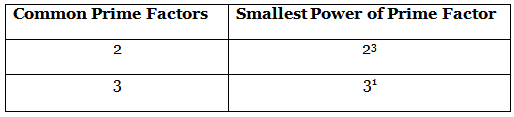HCF (120, 144) = 23 × 3 = 8 × 3 = 24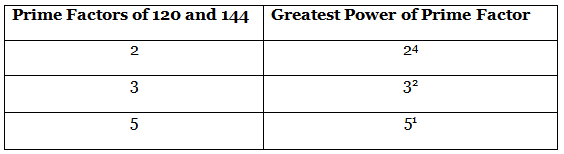LCM is the product of the greatest power of each prime factor involved in the numbers.
LCM (120,144) = 24 × 32 × 5 = 16 × 9 × 5 = 720

Example: If two positive integers p and q can be expressed as p = ab2 and q = a3b, where a, b are prime numbers, find the LCM(p, q).

Sol:

Given: p = ab2 and q = a3b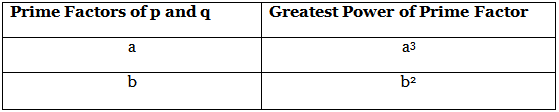LCM is the product of the greatest power of each prime factor involved in the numbers.
LCM(p, q) = a3 × b2 = a3b2

Example: Write the HCF and LCM of the smallest odd composite number and the smallest odd prime number.

Sol:

Smallest odd composite number is 9 and the smallest odd prime number is 3.
9 = 32
3 = 31
Now, the smallest power of the common prime factor is 31 .
HCF (9, 3) = 3
The greatest power of the common prime factor is 32.
LCM (9, 3) = 32 = 9

Example: If HCF (253,440) = 11 and LCM (253,440)= 253 × R. Find the value of R.

Sol:

We know that,
HCF(a, b) × LCM (a, b) = a × b
∴ HCF(253, 440) × LCM (253, 440) = 253 × 440
11 × 253 × R = 253 × 440
R = 253 x 440  / 253 x 11
R = 40

Example: Ravi and Shikha drive around a circular sports field. Ravi takes 16 min to complete one round, while Shikha completes the round in 20 min. If both start at the same point, at the same time and go in the same direction, then after how much time will they meet at the starting point?

Sol:

Time taken by Ravi to drive one round of the circular field = 16 min Time taken by Shikha to drive one round of the circular field =20 min
The time after which they will again meet at the starting point will be equal to the LCM of 16 min and 20 min.
16 = 24
20 = 22 × 5
LCM(16, 20) = 24 × 5 = 16 × 5 = 80
Therefore, Ravi and Shikha will meet again at the starting point after 80 min.

Question for Chapter Notes: Real Numbers
Try yourself:According to the Fundamental Theorem of Arithmetic, which of the following statements is true?

## Revisiting Irrational Numbers

Irrational numbers are those numbers which cannot be written in the form p/q , where p and q are integers and q ≠ 0. E.g.√2, √3, √15

The square roots of all the numbers do not give an irrational number.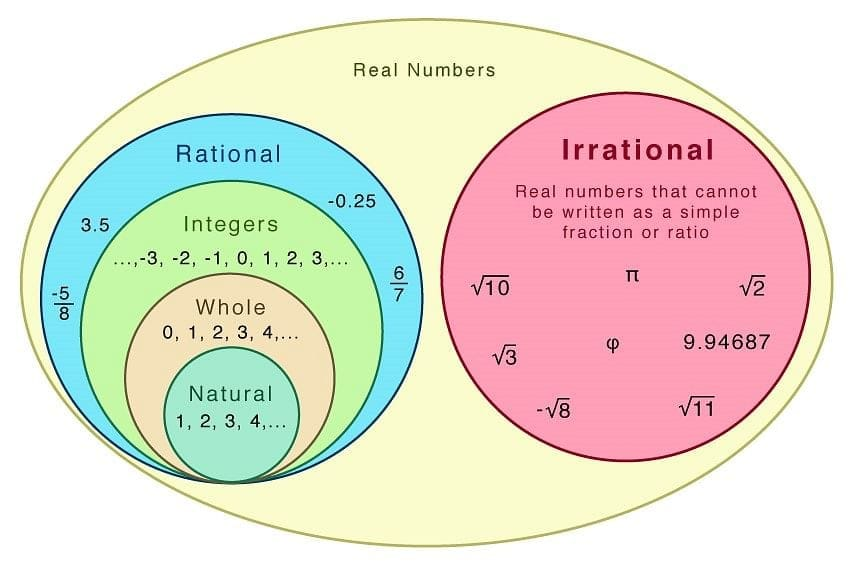For example, √2is an irrational number but √4 = 2, which is rational.
Therefore square roots of all prime numbers are irrational.
If p is a prime number then √p is an irrational number.

Theorem : If a prime number p divides a2, then p divides a, where a is a positive integer.

Proof:
Every positive integer can be expressed as the product of primes.
Let a = p1p2p3 ... ... ... . . pn where p1p2p3 ... ... ... . . pn are all the prime numbers of a.
a2 = (p1p2p3 ... ... ... . . pn)
a2 = (p1p2p3 ... ... ... . . pn)(p1p2p3 ... ... ... . . pn)
a2 = (p12p22p32 ... ... ... . . pn2 )
It is given that p divides a2 . According to the Fundamental Theorem of Arithmetic, we can say that p is one of the prime factors of a2.
According to the Fundamental Theorem of Arithmetic, the prime factorization of a natural number is unique.
Now the only prime factors of a2 are p1p2p3 ... ... ... . . pn. Therefore, p is one of p1p2p3 ... . . pn.
So p is also a factor of a
If p divides a2 then p also divides a.
Let us consider a positive integer 12.
Now, the factors of 12 are 2, 2 and 3.
On squaring 12 we get,
122 = 144
The factors of 144 are 2, 2, 2, 2, 3 and 3.
If one of the factors, let’s say 3 divides 144. Then this factor, 3 will also divide 144, as it is one of the factors of 144 also.

Example: Prove that √2  is an irrational number.

Sol:

Let us assume that √2 is rational. Since it is a rational number it can be expressed in the form a/b  , where a and b are integers and b ≠ 0.
Now, a and b have no common factor other than 1.
√2 = a/b , where a and b are coprime
On squaring both sides, we get
2 = a2 / b2
2b2 = a2
Therefore, 2 divides a2.
We know that when 2 divides a2 then 2 divides a also.
We can write a = 2m, where m is an integer.
Putting a = 2m in 2b2 = a2
2b2 = (2m)2
2b2 = 4m2
b2 = 2m2
Again if 2 divides b2 , then 2 divides b also.
Therefore, 2 is a common factor of a and b.
But this contradicts the fact that a, b have no common factor other than 1.
So, we conclude that √2  is irrational.

Example: Show that 3√2 is an irrational number.

Sol:

Let us assume that 3√2 is rational.
Then 3√2 can be expressed in the form a/b where a and b are integers and b ≠ 0. Now, a and b have no common factor other than 1.
3√2 = a/b , where a and b are coprime integers
√2 = a/3b
Since, 3, a and b are integers, a/3b is rational.
Now, a rational number cannot be equal to an irrational number, that is √2
So, we conclude that 3√2 is irrational.

Example: Show that 5 − √3 is irrational.

Sol:

Let us assume that 5 − √3 is rational. As it is a rational number, it can be expressed in the form a/b where a and b are integers and b ≠ 0. Now, a and b have no common factor other than 1.
5 − √3 = ab, where a and b are coprime
5 − a/b = √3
5b - a = √3
As, 5, a, and b are integers, 5b - a/b  is rational.
Now, √3 is an irrational number and cannot be equal to a rational number.
So, we conclude that 5 − √3 is irrational.

## Euclid’s Division Lemma (Old Syllabus)

A lemma is a proven statement used for proving another statement.

Euclid’s Division Lemma states that any positive integer can be divided by another positive integer in such a way that it leaves a remainder which is smaller than and this is also known as the long division process.
According to Euclid’s Division Lemma, if a and b are two positive integers, there exist unique integers q and r which satisfies the condition, a = bq + r , where 0 ≤ r < b
Here,
a  → Dividend
b → Divisor
q → Quotient
r → Remainder
Dividend = (Divisor Quotient) Remainder.
Let us consider a pair of integers 46 and 7 If we divide 46 by 7,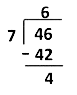Here, 7 is the divisor, 46 is the dividend, 6 is the quotient and 4 is the remainder. Clearly, the remainder, that is 4, is smaller than the divisor, 7.

Example : Show that every positive even integer is of the form 2 q, and that every positive odd integer is of the form 2q + 1, where q is some integer.

Sol:

Let be any positive integer and b =2
According to Euclid’s division lemma there exist and such that
a = bq + r, where 0 ≤ r < b. → (i)
Here, b = 2
Putting  b = 2 in Eq. (i) we get,
a = 2q + r, where q  is any integer
Now, 0 ≤  r < 2
Therefore, the possible remainders are 0 and 1.
When ,r = 0, a = 2q + 0
a = 2q
When r  = 1 , a =2q + 1
If a = 2q , then is an even integer.
If a = 2q , then is an odd integer.
Let us consider an even positive integer, 6 and an odd positive integer, 9.
Then, 6 =2 x 3
and 9 = 2 x 4 + 1
Clearly, the positive even integer is of the form and odd positive integer is of the form 2q +1 .

Example: Show that any positive odd integer is of the form 6q + 1 , or  6q + 3 or 6q + 5, where is any integer.

Sol:

Let be any odd positive integer and b = 6 .
According to Euclid’s division lemma there exist q and r such that
a = bq + r, where  0 ≤ r < b .→ (i)
Putting  b = 6  in Eq.(i) we get,
a=6q + r, where is any integer and 0 6
As 0 ≤ r <6, ∴ r = 0, 1, 2,3, 4,5
When,r = 0 ,a = 6q + 0
r = 1, a = 6q + 1
r = 2, a = 6q + 2
r = 3, a = 6q + 3
r = 4, a = 6q + 4
r = 5, a = 6q + 5
Assuming q=2, then a = 6q = 6 x 2 = 12, an even integer
a = 6q + 2 = 6 x 2 + 2 = 14, an even integer
a = 6q + 4 = 6 x 2 + 4 =16. an even integer.
Since, a is an odd integer a ≠ 6q, a ≠ 6q + 2, a ≠ 6q + 4
Any positive odd integer is of the form 6q+1, 6q + 3, 6q + 5
Now, the value of can q be any integer. Let us assume that the value of q is 2.
Then,6q + 1 = 6 x 2 + 1=13, positive odd integer
6q + 3=6 x 2 + 3 = 12 + 3 = 15, positive odd integer
6q + 5 = 6 x 2 + 5 = 12 + 5 =17, positive odd integer

Example: Show that the square of any positive odd integer is of the form 4q + 1 for some integer q.

Sol:

Let a be any positive integer
According to Euclid’s division lemma there exist q and r such that
a = bq + r, where 0  ≤  r  <  b
Now, is of the form 2m  + 1 , as is a positive odd integer.
When a = 2m + 1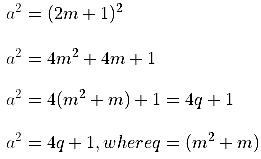Hence, for some integer , the square of any odd integer is of the form 4m + 1
Let us assume that the value of the odd positive integer is 3
Square of 3 = 32 = 9
9 = 2 x 4 + 1
Here, q = 2
Clearly, square of odd integer 3, that is 9, can be written in the form 4q + 1

## Euclid’s Division Algorithm (Old Syllabus)

An algorithm is a series of well-defined steps which gives a procedure to solve a type of problem.

Euclid’s division Algorithm is a technique used to compute the Highest Common Factor (HCF) of two given positive integers. HCF of two positive integers and is the largest  positive integer that divides both and .

To obtain the HCF of two positive integers and , where , we use Euclid’s division Algorithm.

Step 1: Apply Euclid’s division Lemma, to a and b to find whole numbers q and r such that ,a = bq + r, 0  {\color{Blue} \leq }  r  <  b

Step 2: If  r =0, b is the HCF of a and  b . If  r {\color{Blue} \neq } 0 then apply division lemma to b and r .

Step 3: Continue the process till the remainder is zero. The divisor at this stage will be the required HCF.

Example: Use Euclid’s division algorithm, to find the HCF of 870 and 225.

Sol:

Given numbers are 870 and 225.
Here, 870 >225 . By using Euclid’s Division Lemma, a = bq + r, 0  {\color{Blue} \leq }  r  <  b ,
we get,
870 = 225 x 3 +  195
Now, r = 195 ≠ 0  .
We consider new divisor 225 and new remainder 195 and apply the division lemma to get,
225 = 195 x 1  + 30
Again .r =  ≠ 30 0.
We consider new divisor 195 and new remainder 30 and apply the division lemma to get,.
195 = 30 x 6 + 15
Again r = 15  ≠  0.
We consider new divisor 30 and new remainder 15 and apply the division lemma to get,
30 = 15 x 2 +0
Now, the remainder is equal to zero, we stop our procedure, the divisor at this stage is 15. Hence the HCF of 870 and 225 is 15.

Example : Use Euclid’s division algorithm, to find the HCF of 176 and 38220.

Sol:

Given numbers are 176 and 38220.
Here, 38220 > 176 . By using Euclid’s Division Lemma, a = bq + r, 0  ≤  r  <  b , we get,
38220 = 176 x 217 + 28
Now,  r = 28 ≠ 0 .
We consider new divisor 176 and new remainder 28 and apply the division lemma to get,
176 = 28 x 6 + 8
Again r = 8 ≠ 0.
We consider new divisor 28 and new remainder 8 and apply the division lemma to get,
28  =  8 x 3 + 4
r = 4 ≠ 0,
We consider new divisor 8 and new remainder 4 and apply the division lemma to get,
8 = 4 x 2 + 0
Now, the remainder is equal to zero, we stop our procedure, the divisor at this stage is 4. Hence the HCF of 176 and 38220 is 4.

Example: Find the HCF of 180, 252 and 324 by using Euclid’s division Lemma.

Sol:

Given numbers are 180, 252 and 324.
Now, 324 > 252 > 180
We will first find the HCF of 324 and 252
By using Euclid’s Division Lemma, a = bq + r, 0  ≤  r  <  b  , where we get,
324 = 252 x 1 +72
Now,  r = 72 ≠ 0.
We consider now new divisor 252 and new remainder 72 and apply the division lemma to get,
252 = 72 x 3 + 36
Again r = 36 ≠ 0 .
We consider new divisor 72 and new remainder 36 and apply the division lemma to get,
72 = 36 x  2 + 0.
The remainder is equal to zero, the divisor at this stage is 36. Hence the HCF of 324 and 252 is 36.
Now, we will use Euclid’s Division Lemma for 180 and 36 to get,
180 = 36 x5 +0
The HCF of 180 and 36 is 36.
Hence the HCF of 324, 252 and 180 is 36.

Example : Two tanks contain 504 L and 735 L of milk, respectively. Find the maximum capacity of a container which can measure the milk of either tank in exact number of  times.

Sol:

The capacities of the two tanks are 504L and 735L.
The maximum capacity of a container will be the HCF of 504 and 735.
735 > 504
By using Euclid’s Division Lemma we get,
735 = 504 x 1 + 231
Now, r  =  231 ≠ 0 .
We will consider new divisor 504 and new remainder 231 and apply Euclid’s Division Lemma to get,
504 = 231 x 2 +42
Again, r = 42 ≠ 0.
We now consider new divisor 231 and new remainder 42, and apply Euclid’s Division Lemma to get,
231  = 42 x 5 =21
r  =  21 ≠ 0.
We now consider new divisor 42 and new remainder 21, and apply Euclid’s Division Lemma to get,
42 = 21 x 2 + 0
The remainder is equal to zero, the divisor at this stage is 21. Hence the HCF of 504 and 735 is 21.
Therefore, the maximum capacity of the required container is 21L.

Example : Three pieces of timber 42 m, 49 m and 56 m long have to be divided into planks of the same length. What is the greatest possible length of each plank?

Sol:

The greatest possible length of the plank will be the HCF of 42m, 49 m and 56 m.
Now, 56 > 49 > 42
We will first find the HCF of 56 and 49.
By using Euclid’s Division Lemma we get,
56 = 49 x 1 +7
Now, r  =7 ≠ 0 .
We will consider new divisor 49 and new remainder 7 and apply Euclid’s Division Lemma to get,
49 = 7 x 7 +  0
The remainder is equal to zero, the divisor at this stage is 7. Hence the HCF of 56 and 49 is 7.
Now, using Euclid’s Division Lemma for 42 and 7 we get,
42 =  7 x 6 + 0
As the remainder is 0, the divisor in this case is the HCF of 42 and 7.
Therefore, the HCF of 42 and 7 is 7.
The HCF of and is 7.
Hence the greatest possible length of the plank is 7m

## Revisiting Rational Numbers and their Decimal Expansion (Old Syllabus)

Revisiting Rational Numbers

Rational Numbers and their Decimal Expansion
The decimal expansion of every rational number is either terminating or non-terminating repeating.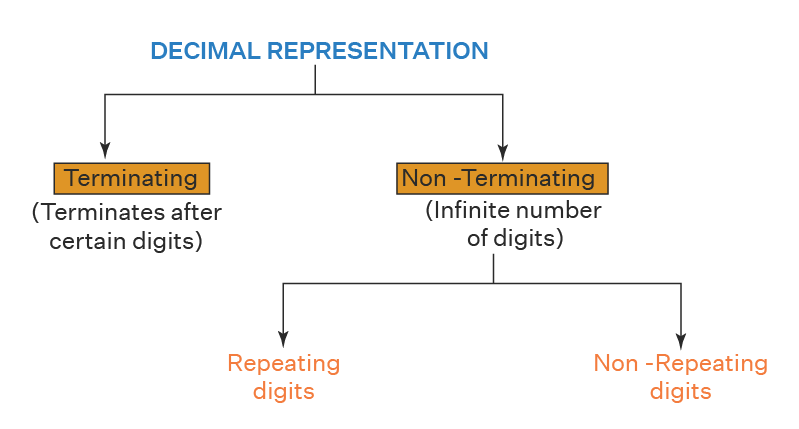Terminating Decimal Expansion
The number which terminates after a finite number of steps in the process of division is called terminating decimal expansion. E.g. 6.25, 1.14

Non-Terminating Decimal Expansion
The number which does not terminate in the process of division is called non-terminating decimal expansion.
There are two types of non-terminating decimal expansions
(a) Non-terminating repeating Decimal Expansion
The number which does not terminate but repeats the particular number again and again in the process of division is said to be non-terminating repeating decimal. E.g. 0.333333
(b) Non-terminating Non-repeating Decimal Expansion
The number which neither terminates nor repeats the particular number in the process of division is said to be non-terminating repeating decimal. E.g. 1.03303033

Theorem 1:
Let x be a rational number whose decimal expansion terminates.
Then x can be expressed in the form p/q , where p and q are coprime and the prime factorization of q is of the form 2m5n , where m, n are non- negative integers.

Theorem 2:
Let x = p/q be a rational number, such that the prime factorization of q is of the form 2m5n , where m, n are non-negative integers, then p/q has a terminating decimal.
23/80 = 23 / 24 x 5
Multiplying both numerator and denominator by 53
= 23 x 53 / 24 x 5 x 53 = 23 x 53 /24 x 54
2875/(2 x 5)4
= 2875/104
= 2875/10000
= 0.2875

Theorem 3:
Let x = p/q be a rational number, such that the prime factorization of q is not of the form 2m5n , where m, n are non-negative integers, then x has decimal expansion which is non-terminating repeating.
Example: Without actually performing the long division, state whether 543/225  has a terminating decimal expansion or non-terminating recurring decimal expansion.

Sol:

543/225 = 181/75 = 181/3 x 52
Since the factors of the denominator (3.5.2) is not of the form 2m5n .
So, 543/225 is non-terminating recurring decimal expansion.

Example: The decimal expansion of the rational number 53/24 x 53 will terminate after how many places of decimal.

Sol:

53/24 x 53
Now, multiplying both numerator and denominator by 5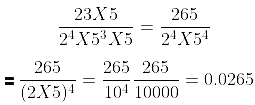The decimal expansion of the rational number 53/24 x 53 will terminate after 4 places of decimal.

Example: If 299/4000 = 299/{2m5n, then find the value of m and n, where m and n are non- negative integers. Hence, write its decimal expansion without actual division.

Sol: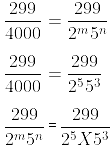m = 5 and n = 3
Now, 299/4000 can be expressed as 299/25 x 53
We have, 299/25 x 53
Multiplying both numerator and denominator by {\color{Blue} 5^{2}}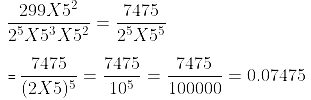Example: What can you say about the prime factorization of the denominators of the following rational:
(i) 42.123456789
(ii)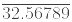Sol:

(i) Since 42.123456789 has a terminating decimal expansion. So, its denominator is of the form 2m5n , where m and n are non-  negative integers.
(ii) Since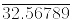has non-terminating decimal expansion. So, its denominator has factors other than 2 or 5.

The document Real Numbers Chapter Notes | Mathematics (Maths) Class 10 is a part of the Class 10 Course Mathematics (Maths) Class 10.
All you need of Class 10 at this link: Class 10

## Mathematics (Maths) Class 10

115 videos|478 docs|129 tests

## FAQs on Real Numbers Chapter Notes - Mathematics (Maths) Class 10

 1. What are real numbers?Ans. Real numbers are numbers that can be represented on the number line. They include rational numbers (numbers that can be expressed as a fraction) and irrational numbers (numbers that cannot be expressed as a fraction).
 2. What is the fundamental theorem of arithmetic?Ans. The fundamental theorem of arithmetic states that every positive integer greater than 1 can be expressed as a unique product of prime numbers, up to the order of the factors.
 3. What is Euclid's division lemma?Ans. Euclid's division lemma states that for any two positive integers a and b, there exist unique integers q and r such that a = bq + r, where 0 ≤ r < b. Here, q is the quotient and r is the remainder.
 4. What is the difference between Euclid's division lemma and Euclid's division algorithm?Ans. Euclid's division lemma is a mathematical statement that provides a theoretical foundation for the Euclidean algorithm. On the other hand, Euclid's division algorithm is a step-by-step procedure based on the division lemma to find the highest common factor (HCF) of two positive integers.
 5. How are rational numbers and their decimal expansion related?Ans. Rational numbers can be expressed as a fraction of two integers. Their decimal expansion can be either terminating (ends after a finite number of digits) or recurring (repeats a pattern of digits). For example, 1/2 can be written as 0.5, which is a terminating decimal, while 1/3 can be written as 0.333..., which is a recurring decimal.

## Mathematics (Maths) Class 10

115 videos|478 docs|129 testsExplore Courses for Class 10 examSignup to see your scores go up within 7 days! Learn & Practice with 1000+ FREE Notes, Videos & Tests.
10M+ students study on EduRev
Track your progress, build streaks, highlight & save important lessons and more!
Related Searches

,

,

,

,

,

,

,

,

,

,

,

,

,

,

,

,

,

,

,

,

,

;